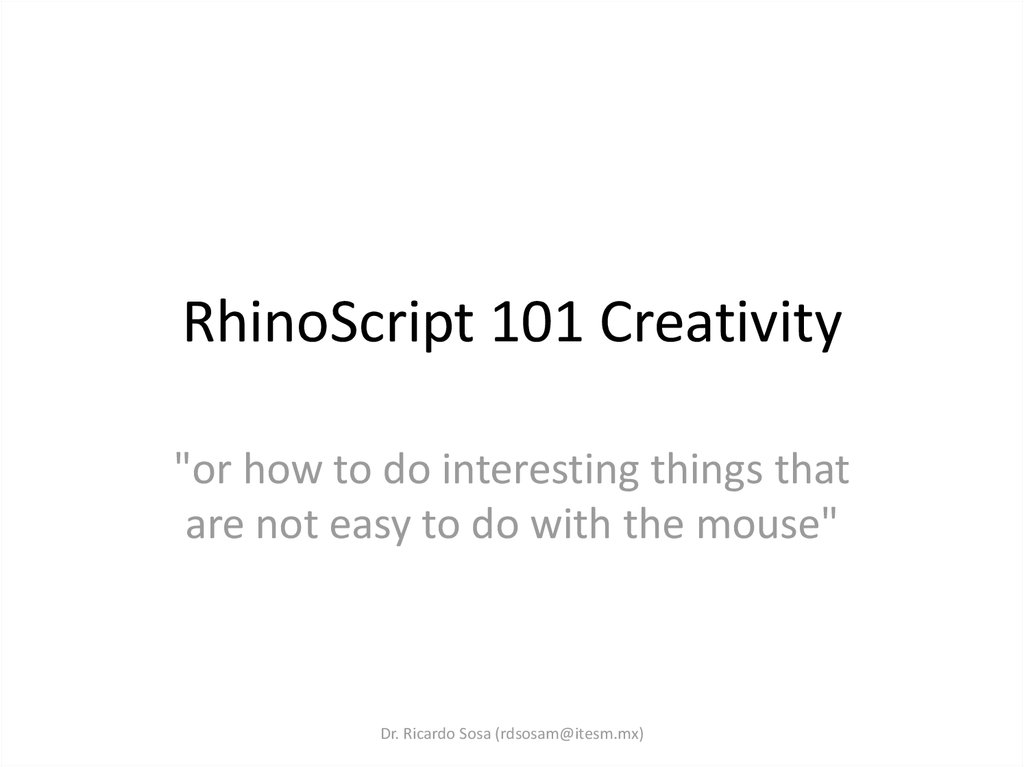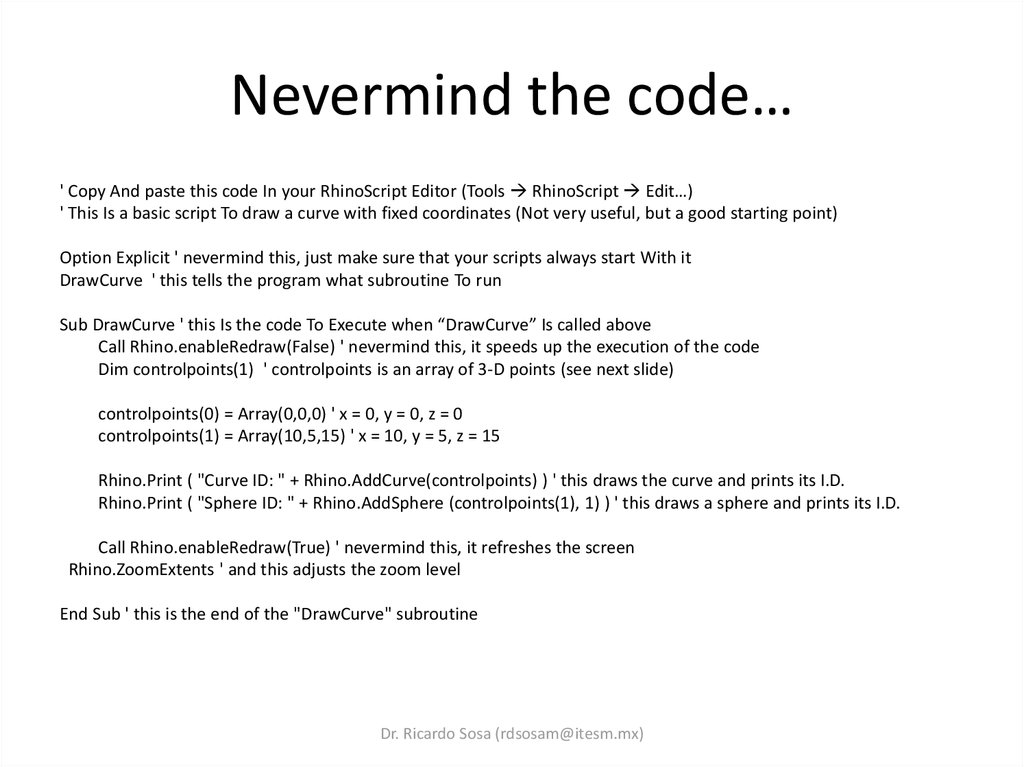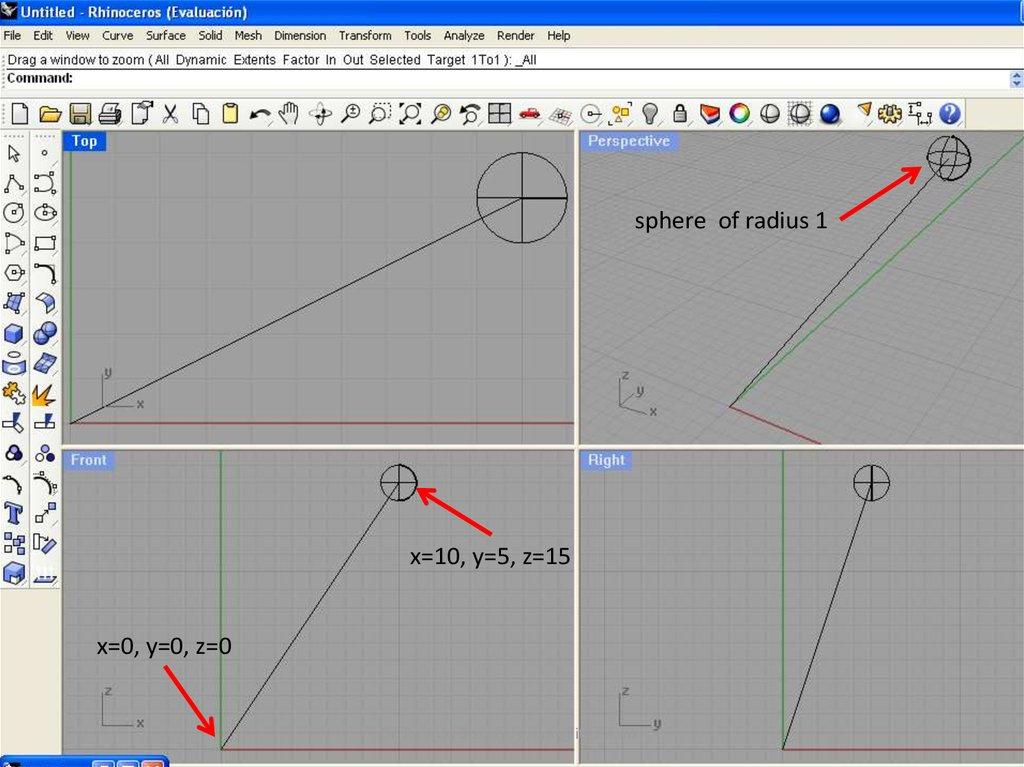# RhinoScript 101 Creativity "or how to do interesting things that are not easy to do with the mouse"

## 1. RhinoScript 101 Creativity

"or how to do interesting things that
are not easy to do with the mouse"
Dr. Ricardo Sosa ([email protected])

## 2. Nevermind the code…

' Copy And paste this code In your RhinoScript Editor (Tools RhinoScript Edit…)
' This Is a basic script To draw a curve with fixed coordinates (Not very useful, but a good starting point)
DrawCurve ' this tells the program what subroutine To run
Sub DrawCurve ' this Is the code To Execute when “DrawCurve” Is called above
Call Rhino.enableRedraw(False) ' nevermind this, it speeds up the execution of the code
Dim controlpoints(1) ' controlpoints is an array of 3-D points (see next slide)
controlpoints(0) = Array(0,0,0) ' x = 0, y = 0, z = 0
controlpoints(1) = Array(10,5,15) ' x = 10, y = 5, z = 15
Rhino.Print ( "Curve ID: " + Rhino.AddCurve(controlpoints) ) ' this draws the curve and prints its I.D.
Rhino.Print ( "Sphere ID: " + Rhino.AddSphere (controlpoints(1), 1) ) ' this draws a sphere and prints its I.D.
Call Rhino.enableRedraw(True) ' nevermind this, it refreshes the screen
Rhino.ZoomExtents ' and this adjusts the zoom level
End Sub ' this is the end of the "DrawCurve" subroutine
Dr. Ricardo Sosa ([email protected])

## 3. Diapositiva 3

x=10, y=5, z=15
x=0, y=0, z=0
Dr. Ricardo Sosa ([email protected])

## 4. Diapositiva 4

controlpoints()
controlpoints(0)
controlpoints(1)
x0
y0
z0
x1
y1
z1
0
0
0
10
5
15
Dr. Ricardo Sosa ([email protected])

## 5. Hold on! What's an "array"?

Hold on! What's an "array"?
Dr. Ricardo Sosa ([email protected])

## 6. An array is like a box

Dr. Ricardo Sosa ([email protected])

## 7. A box that holds 3D coordinates

Dr. Ricardo Sosa ([email protected])

## 8. Diapositiva 8

' Copy and paste this code in your RhinoScript Editor (Tools RhinoScript Edit…)
' This is a basic script to draw a curve with fixed coordinates (Not very useful, but a good starting point)
DrawCurve ' this tells the program what subroutine to run
Sub DrawCurve ' this is the code to run when “DrawCurve” is called above
Call Rhino.enableRedraw(False) ' nevermind this, it speeds up the execution of the code
Dim controlpoints(1) ' controlpoints is an array of 3-D points (see next slide)
controlpoints(0) = Array(0,0,0) ' x = 0, y = 0, z = 0
controlpoints(1) = Array(10,5,15) ' x = 10, y = 5, z = 15
Rhino.Print ( "Curve ID: " + Rhino.AddCurve(controlpoints) ) ' this draws the curve and prints its I.D.
Rhino.Print ( "Sphere ID: " + Rhino.AddSphere (controlpoints(1), 1) ) ' this draws a sphere and its I.D.
Call Rhino.enableRedraw(True) ' nevermind this, it refreshes the screen
Rhino.ZoomExtents ' and this adjusts the zoom level
End Sub ' this is the end of the "DrawCurve" subroutine
Dr. Ricardo Sosa ([email protected])

## 9. Diapositiva 9

Curve ID: 2312ea39-2894-4d1f-b31e-406fa88e5824
Sphere ID: 19ea41fa-0e4f-4514-b701-36c39939113a
Dr. Ricardo Sosa ([email protected])

## 10. Now some randomness…

' Copy and paste this code in your RhinoScript Editor (Tools RhinoScript Edit…)
' This is a basic script to draw a curve with fixed coordinates (Not very useful, but a good starting point)
DrawCurve ' this tells the program what subroutine to run
Sub DrawCurve ' this is the code to run when “DrawCurve” is called above
Call Rhino.enableRedraw(False) ' nevermind this, it speeds up the execution of the code
Dim controlpoints(1) ' controlpoints is an array of 3-D points (see next slide)
controlpoints(0) = Array(0,0,0) ' x = 0, y = 0, z = 0
controlpoints(1) = Array(randomBetween(-10,10),randomBetween(-10,10),15) ' x = random, y = random, z = 15
Rhino.Print ( "Curve ID: " + Rhino.AddCurve(controlpoints) ) ' this draws the curve and prints its I.D.
Rhino.Print ( "Sphere ID: " + Rhino.AddSphere (controlpoints(1), 1) ) ' this draws a sphere and its I.D.
Call Rhino.enableRedraw(True) ' nevermind this, it refreshes the screen
Rhino.ZoomExtents ' and this adjusts the zoom level
End Sub ' this is the end of the "DrawCurve" subroutine
Function randomBetween(min,max) ' this is the code to generate random numbers between limits
randomBetween = Rnd*(max-min)+min ' returns a random number between the limits specified
End Function ' end of the randomness function
Dr. Ricardo Sosa ([email protected])

## 11. Diapositiva 11

Ricardo
Sosa a
([email protected])
After runningDr.the
code
few times you get something like this…

## 12. Now some recursion…

' Copy and paste this code in your RhinoScript Editor (Tools RhinoScript Edit…)
' This is a basic script to draw a curve with fixed coordinates (Not very useful, but a good starting point)
DrawCurve ' this tells the program what subroutine to run
Sub DrawCurve ' this is the code to run when “DrawCurve” is called above
Call Rhino.enableRedraw(False) ' nevermind this, it speeds up the execution of the code
Dim controlpoints(1) ' controlpoints is an array of 3-D points (see next slide)
Dim i
For i=0 To 100
controlpoints(0) = Array(0,0,0) ' x = 0, y = 0, z = 0
controlpoints(1) = Array(randomBetween(-10,10),randomBetween(-10,10),15) ' x = random, y = random, z = 15
Rhino.AddCurve controlpoints ' this draws the curve
Rhino.AddSphere controlpoints(1), 1 ' this draws a sphere
Next
Call Rhino.enableRedraw(True) ' nevermind this, it refreshes the screen
Rhino.ZoomExtents ' and this adjusts the zoom level
End Sub ' this is the end of the "DrawCurve" subroutine
Function randomBetween(min,max) ' this is the code to generate random numbers between limits
randomBetween = Rnd*(max-min)+min ' returns a random number between the limits specified
End Function ' end of the randomness function
Dr. Ricardo Sosa ([email protected])

## 13. Diapositiva 13

Dr. Ricardo Sosa ([email protected])

## 14. Diapositiva 14

controlpoints()
controlpoints(0)
controlpoints(1)
x0
y0
z0
x1
y1
z1
0
0
0
0
4
15
0
0
0
-4
6
15
0
0
0
-3
-9
15
0
0
0
6
-6
15
0
0
0
-1
-6
15
0
0
0
-2
9
15
0
0
0
10
-9
15
0
0
0
-2
-7
15
0
0
0
-10
-2
15
Dr. Ricardo Sosa ([email protected])
0
0
0
-4
9
15

## 15. Diapositiva 15

Dr. Ricardo
Sosa ([email protected])
One
thousand
times… (slooow!)

## 16. More interesting curves…

' Copy and paste this code in your RhinoScript Editor (Tools RhinoScript Edit…)
' This is a basic script to draw a curve with fixed coordinates (Not very useful, but a good starting point)
DrawCurve ' this tells the program what subroutine to run
Sub DrawCurve ' this is the code to run when “DrawCurve” is called above
Call Rhino.enableRedraw(False) ' nevermind this, it speeds up the execution of the code
Dim controlpoints(2), i ' controlpoints is an array of 3-D points (see next slide)
For i=0 To 50
controlpoints(0) = Array(0,0,0) ' x = 0, y = 0, z = 0
controlpoints(1) = Array(randomBetween(-5,5),randomBetween(-5,5),0)
controlpoints(2) = Array(randomBetween(-10,10),randomBetween(-10,10),15)
Rhino.AddCurve controlpoints, 2 ' this draws the curve of two degrees now
Rhino.AddSphere controlpoints(1), 0.25 ' this draws a small sphere at second point
Next
Call Rhino.enableRedraw(True) ' nevermind this, it refreshes the screen
Rhino.ZoomExtents ' and this adjusts the zoom level
End Sub ' this is the end of the "DrawCurve" subroutine
Function randomBetween(min,max) ' this is the code to generate random numbers between limits
randomBetween = Rnd*(max-min)+min ' returns a random number between the limits specified
End Function ' end of the randomness function
Dr. Ricardo Sosa ([email protected])

## 17. Diapositiva 17

Dr. Ricardo Sosa ([email protected])

## 18. Diapositiva 18

Select any line and press F10:
You will notice that the small spheres are drawn at the second control point…
Dr. Ricardo Sosa ([email protected])

## 19. Diapositiva 19

' Copy and paste this code in your RhinoScript Editor (Tools RhinoScript Edit…)
' This is a basic script to draw a curve with fixed coordinates (Not very useful, but a good starting point)
DrawCurve ' this tells the program what subroutine to run
Sub DrawCurve ' this is the code to run when “DrawCurve” is called above
Call Rhino.enableRedraw(False) ' nevermind this, it speeds up the execution of the code
Dim controlpoints(2), i ' controlpoints is an array of 3-D points (see next slide)
For i=0 To 50
controlpoints(0) = Array(0,0,0) ' x = 0, y = 0, z = 0
controlpoints(1) = Array(randomBetween(-5,5),randomBetween(-5,5),0)
controlpoints(2) = Array(randomBetween(-10,10),randomBetween(-10,10),15)
Rhino.AddCurve controlpoints, 2 ' this draws the curve
Rhino.AddSphere controlpoints(1), 0.25 ' this draws a small sphere at second point
Rhino.AddSphere controlpoints(2), 0.75 ' this draws a big sphere at third point
Next
Call Rhino.enableRedraw(True) ' nevermind this, it refreshes the screen
Rhino.ZoomExtents ' and this adjusts the zoom level
End Sub ' this is the end of the "DrawCurve" subroutine
Function randomBetween(min,max) ' this is the code to generate random numbers between limits
randomBetween = Rnd*(max-min)+min ' returns a random number between the limits specified
End Function ' end of the randomness function
Dr. Ricardo Sosa ([email protected])

## 20. Diapositiva 20

Dr. Ricardo Sosa ([email protected])

## 21. Time for a challenge…

How do you achieve the following?
Dr. Ricardo Sosa ([email protected])

## 22. Diapositiva 22

Dr. Ricardo Sosa ([email protected])

## 23. Diapositiva 23

' Copy and paste this code in your RhinoScript Editor (Tools RhinoScript Edit…)
' This is a basic script to draw a curve with fixed coordinates (Not very useful, but a good starting point)
DrawCurve ' this tells the program what subroutine to run
Sub DrawCurve ' this is the code to run when “DrawCurve” is called above
Call Rhino.enableRedraw(False) ' nevermind this, it speeds up the execution of the code
Dim controlpoints(3), i ' controlpoints is an array of 3-D points (see next slide)
For i=0 To 50
controlpoints(0) = Array(0,0,0) ' x = 0, y = 0, z = 0
controlpoints(1) = Array(randomBetween(-5,5),randomBetween(-5,5),0)
controlpoints(2) = Array(randomBetween(-10,10),randomBetween(-10,10),15)
controlpoints(3) = Array(randomBetween(-10,10),randomBetween(-10,10),20)
Rhino.AddCurve controlpoints, 3 ' this draws the curve
Rhino.AddSphere controlpoints(1), 0.25 ' this draws a small sphere at second point
Rhino.AddSphere controlpoints(2), 0.25 ' this draws a big sphere at third point
Rhino.AddSphere controlpoints(3), 0.75 ' this draws a big sphere at third point
Next
Call Rhino.enableRedraw(True) ' nevermind this, it refreshes the screen
Rhino.ZoomExtents ' and this adjusts the zoom level
End Sub ' this is the end of the "DrawCurve" subroutine
Function randomBetween(min,max) ' this is the code to generate random numbers between limits
randomBetween = Rnd*(max-min)+min ' returns a random number between the limits specified
End Function ' end of the randomness function
Dr. Ricardo Sosa ([email protected])

## 24. Diapositiva 24

Dr. Ricardo Sosa ([email protected])

## 25. Add another set of coordinates…

Dr. Ricardo Sosa ([email protected])

## 26. Rhino.Command "anycommand"

Rhino.Command "anycommand"
Shift + Rightclick any tool icon to see
its _Command
Dr. Ricardo Sosa ([email protected])

## 27. Diapositiva 27

Dr. Ricardo Sosa ([email protected])

## 28. Diapositiva 28

' Copy and paste this code in your RhinoScript Editor (Tools RhinoScript Edit…)
' This is a basic script to draw a curve with fixed coordinates (Not very useful, but a good starting point)
DrawCurve ' this tells the program what subroutine to run
Sub DrawCurve ' this is the code to run when “DrawCurve” is called above
Call Rhino.enableRedraw(False) ' nevermind this, it speeds up the execution of the code
Dim controlpoints(3), i ' controlpoints is an array of 3-D points (see next slide)
Dim strCmd, curveID
For i=0 To 50
controlpoints(0) = Array(0,0,0) ' x = 0, y = 0, z = 0
controlpoints(1) = Array(randomBetween(-5,5),randomBetween(-5,5),0)
controlpoints(2) = Array(randomBetween(-10,10),randomBetween(-10,10),15)
controlpoints(3) = Array(randomBetween(-10,10),randomBetween(-10,10),20)
curveID = Rhino.AddCurve(controlpoints, 3) ' this draws the curve
Rhino.SelectObject(curveID)
Rhino.Command "_Pipe " & 1.0 & " Enter " & 1.0 & " Enter"
Next
Call Rhino.enableRedraw(True) ' nevermind this, it refreshes the screen
Rhino.ZoomExtents ' and this adjusts the zoom level
End Sub ' this is the end of the "DrawCurve" subroutine
Function randomBetween(min,max) ' this is the code to generate random numbers between limits
randomBetween = Rnd*(max-min)+min ' returns a random number between the limits specified
End Function ' end of the randomness function
Dr. Ricardo Sosa ([email protected])

## 29. Diapositiva 29

Dr. Ricardo Sosa ([email protected])

## 30. Diapositiva 30

Dr. Ricardo Sosa ([email protected])

## 31. Diapositiva 31

Dr. Ricardo Sosa ([email protected])

## 32. Diapositiva 32

After you add a curve, select it with:
Rhino.SelectObject(curveID)
Then apply the command:
Rhino.Command "_Pipe " & 1.0 & " Enter "
& 1.0 & " Enter"
Dr. Ricardo Sosa ([email protected])

## 33. Due next class…

Do something interesting of your
own!
Dr. Ricardo Sosa ([email protected])